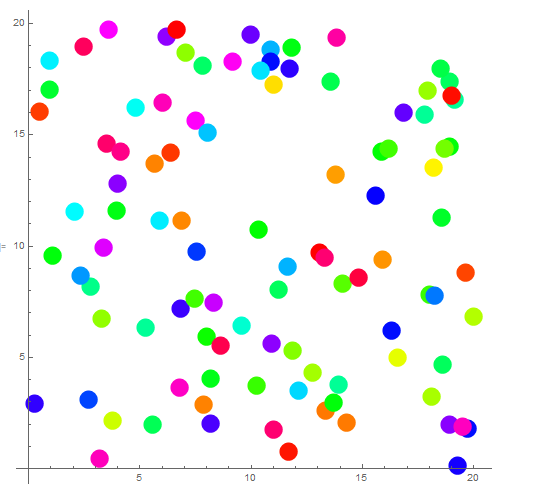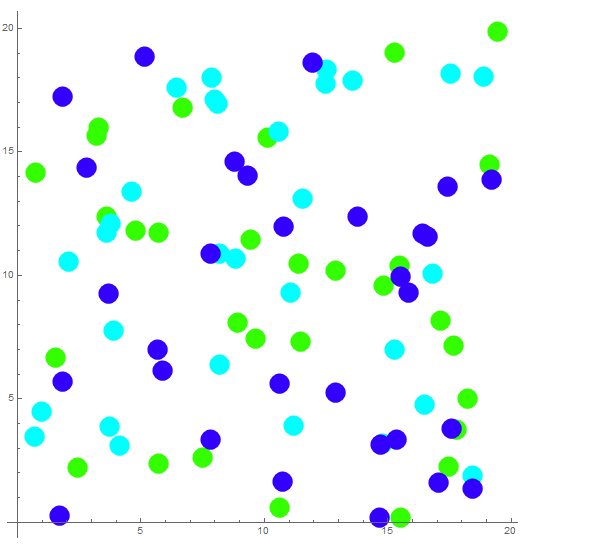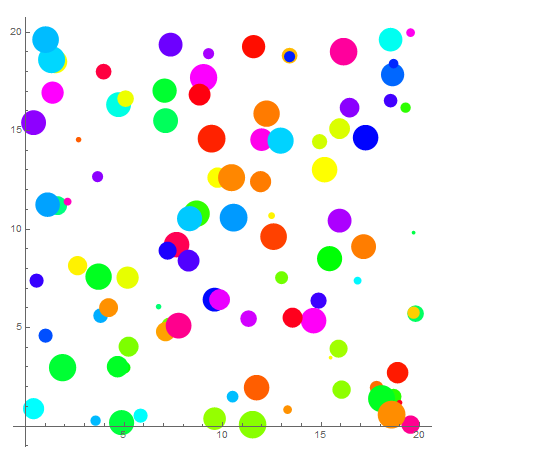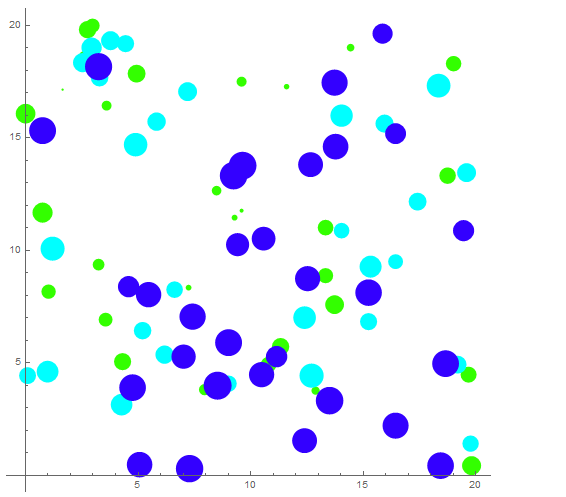# Mathematica散点图美化2018年3月26日06:02:32

2011字阅读6分42秒

## 增加散点的色彩

``````(*pts中的数据类似{名字,横座标,纵座标,大小,随机数(用来控制最后的颜色)}*)
pts = Table[{"Name" <> ToString[i], RandomReal, RandomReal,  17, RandomReal[]}, {i, 100}];
(*画出圆盘的位置和对应的大小*)
fp[{name_, x_, y_, size_, c_}] := Style[Disk[{x, y}, Sqrt[size]/10], Hue[c]];
(*对pts中每个数据做fp函数的作用*)
Graphics[fp /@ pts, Axes -> True, AxesOrigin -> {0, 0}]````````````(*pts中的数据类似{名字,横座标,纵座标,大小,随机数(用来控制最后的颜色)}*)
pts1 = Table[{"Name" <> ToString[i], RandomReal, RandomReal, 17, .3}, {i, 30}];
pts2 = Table[{"Name" <> ToString[i], RandomReal, RandomReal, 17, .5}, {i, 30}];
pts3 = Table[{"Name" <> ToString[i], RandomReal, RandomReal, 17, .7}, {i, 30}];
(*画出圆盘的位置和对应的大小*)
fp[{name_, x_, y_, size_, c_}] := Style[Disk[{x, y}, Sqrt[size]/10], Hue[c]];
(*对pts中每个数据做fp函数的作用*)
Graphics[fp /@ (Flatten[{pts1, pts2, pts3}, 1]), Axes -> True,  AxesOrigin -> {0, 0}]``````## 增加散点的大小

``````(*pts中的数据类似{名字,横座标,纵座标,大小,随机数(用来控制最后的颜色)}*)
pts = Table[{"Name" <> ToString[i], RandomReal, RandomReal,  RandomInteger, RandomReal[]}, {i, 100}];
(*画出圆盘的位置和对应的大小*)
fp[{name_, x_, y_, size_, c_}] :=  Style[Disk[{x, y}, Sqrt[size]/10], Hue[c]];
(*对pts中每个数据做fp函数的作用*)
Graphics[fp /@ pts, Axes -> True, AxesOrigin -> {0, 0}]````````````(*pts中的数据类似{名字,横座标,纵座标,大小,随机数(用来控制最后的颜色)}*)
pts1 = Table[{"Name" <> ToString[i], RandomReal, RandomReal,  RandomReal, .3}, {i, 30}];
pts2 = Table[{"Name" <> ToString[i], RandomReal, RandomReal,  RandomReal[{10, 30}], .5}, {i, 30}];
pts3 = Table[{"Name" <> ToString[i], RandomReal, RandomReal,  RandomReal[{20, 40}], .7}, {i, 30}];
(*画出圆盘的位置和对应的大小*)
fp[{name_, x_, y_, size_, c_}] :=   Style[Disk[{x, y}, Sqrt[size]/10], Hue[c]];
(*对pts中每个数据做fp函数的作用*)
Graphics[fp /@ (Flatten[{pts1, pts2, pts3}, 1]), Axes -> True,  AxesOrigin -> {0, 0}]``````• 微信公众号
• 关注微信公众号
•• QQ群
• 我们的QQ群号
•• 本文由 发表于 2018年3月26日06:02:32
• 转载请务必保留本文链接：https://mathpretty.com/9087.html